# Print Versus Write

The reason you might choose a PrintStream instead of a raw OutputStream is for its print( ) and println( ) methods. These methods each convert their argument to a String and then convert the String to bytes in a specific encoding before writing it to the underlying output stream. For example, consider this PrintStream connected to a file named numbers.dat:

```PrintStream out = new PrintStream(new FileOutputStream("numbers.dat"));
```

Suppose we use a simple for loop to write the numbers from 0 to 127 in that file:

```for (int i = 0; i <= 127; i++) out.write(i);
```

When we're done, the file contains 128 bytes: the first byte is 0, the second is 1, the third is 2, and so on. It's pure binary data. If you try to open it up in a text editor you'll see goop, as shown in Figure 7-1. Some of those binary numbers happen to be interpretable as ASCII text, but that's an accident. They're really just bytes. Many of them aren't printable.

Figure 7-1. A binary file in a text editor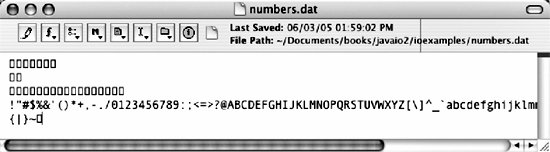Now suppose instead of using the write( ) method we use the print( ) method:

```for (int i = 0; i <= 127; i++) out.print(i);
```

This time the PrintStream does not write raw binary data in the file. Instead, it converts each number into its ASCII string equivalent and writes that string. For instance, instead of writing the byte 20, it writes the character 2 followed by the character 0. If you open the file in a text editor now, you'll see something like the screenshot shown in Figure 7-2.

Figure 7-2. A text file in a text editor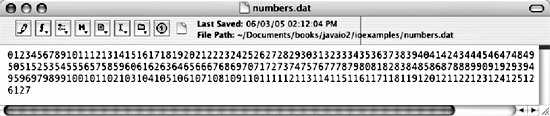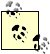It's not absolutely guaranteed that the string will be written in ASCII. On a few IBM mainframes, EBCDIC might be used instead. However, given the range of characters used here, it's pretty likely that the resulting file will make sense when interpreted as ASCII. More on this point shortly.

The println( ) method prints a platform-specific line terminator after printing its argument. Suppose instead of using the print( ) method we use the println( ) method:

```for (int i = 0; i <= 127; i++) out.println(i);
```

Then the output is even neater, as shown in Figure 7-3.

Figure 7-3. A text file with line breaks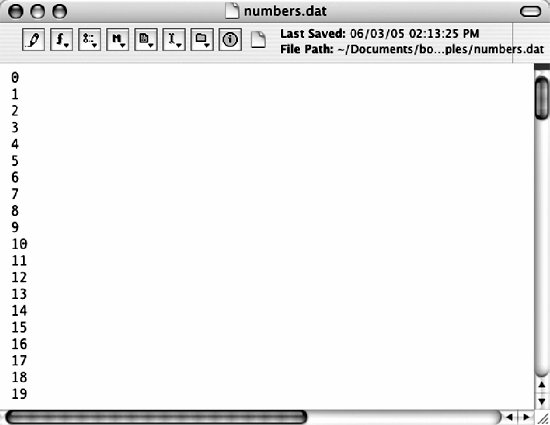These examples used ints, but the PrintStream class has print( ) and println( ) methods for every Java data type. The method signatures are:

```public void print(boolean b)
public void print(char c)
public void print(int i)
public void print(long l)
public void print(float f)
public void print(double d)
public void print(char[] s)
public void print(String s)
public void print(Object o)
public void println(boolean b)
public void println(char c)
public void println(int i)
public void println(long l)
public void println(float f)
public void println(double d)
public void println(char[] s)
public void println(String s)
public void println(Object o)
```

You can pass anything at all to a print( ) method. Whatever argument you supply is guaranteed to match at least one of these methods. Object types are converted to strings by invoking their toString( ) methods. Primitive types are converted with the appropriate String.valueOf( ) methods.

One aspect of making System.out simple for quick jobs is not in the PrintStream class at all, but in the compiler. Because Java overloads the + operator to signify concatenation of strings, primitive data types, and objects, you can pass multiple variables to the print( ) and println( ) methods, which are then converted to strings and concatenated. For example, consider the line:

```System.out.println("As of " + (new Date( )) + " there have been over "
+ hits + " hits on the web site." );
```

The compiler rewrites this complicated expression as:

```StringBuffer sb = new StringBuffer( );
sb.append("As of ");
Date d = new Date( );
sb.append(d);
sb.append(" there have been over ");
sb.append(hits);
sb.append(" hits on the web site.")
String s = sb.toString( );
System.out.println(s);
```

The StringBuffer append( ) method is overloaded in much the same way that the print( ) and println( ) methods are; as a result, it can handle any Java data type.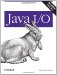Java I/O
ISBN: 0596527500
EAN: 2147483647
Year: 2004
Pages: 244